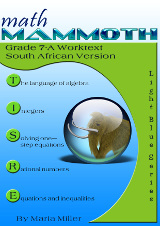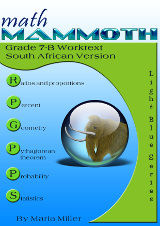^

You are here: HomeSouth African version → Grade 7

# Math Mammoth Grade 7, South African version

Math Mammoth Grade 7, South African Version is a complete maths program for 7th grade. It consists of two student worktexts (A and B), a separate answer key for each worktext, tests, mixed revisions, and in the download version, an additional worksheet maker (Internet access required) to make extra practice worksheets when needed.227 pages
202 lesson pages288 pages
253 lesson pages

## Prices & ordering

(part A and B worktexts, answer keys, tests, mixed revisions, a worksheet maker, and Soft-Pak)

The two books (worktext part A and part B) deal with

• introduction to basic algebra concepts
• integers and their operations
• solving one-step equations, including with negative numbers
• operations with negative rational numbers
• solving linear equations and writing equations for word problems
• graphing linear equations and an introduction to the concept of slope
• ratios, rates, proportions, and percent
• geometry: angle relationships, compass & ruler constructions, drawing problems, Pi and the area and circumference of a circle, cross-sections formed when cutting solids, surface area, and volume
• the Pythagorean theorem
• probability
• statistics

Please also see the table of contents for 6-A and 6-B (in the sample files), which will let you see the topics covered in more detail.

Some main features of Math Mammoth complete curriculum (Light Blue series) are:

• focuses on understanding of mathematical concepts
• uses clear explanations, lots of visual exercises and pattern exercises
• mastery oriented: concentrates at length on a topic, with very few topics per grade
• emphasizes mental maths and developing number sense
• very little teacher preparation needed

The downloadable version includes a worksheet maker (Internet access required) that lets you make extra practice worksheets for many of the topics in the curriculum.

The PDFs in the download version are enabled for annotation. This means that if you prefer, the student can fill them in on the computer, using the typewriter and drawing tools in Acrobat Reader version 9 or greater.

## Introduction

Math Mammoth Grade 7-A and Grade 7-B worktexts comprise a complete math curriculum for seventh grade mathematics studies. This is a pre-algebra course, and students can continue to an algebra 1 curriculum after studying it.

We start out with an introduction to basic algebra, which is in many ways a review of the same topics from 6th grade. The first chapter reviews the order of operations, the concepts of an expression and equation, and the distributive property. Students learn about the commutative and associative properties of addition and multiplication, and they simplify expressions that don’t involve negative numbers.

In chapter 2, we study integers and their operations in detail. Some of this is, again, review from 6th grade, and some of it is new. The four operations of integers are explained with the help of two visual models: the number line and counters, hopefully providing an intuitive understanding of the processes. Students need to be able to add, subtract, multiply, and divide integers when they learn to solve equations in chapter 3. In the end of the chapter students also learn about negative fractions.

The lesson about distance contains a formula that may look unfamiliar to the teacher. You can find the distance between two integers by taking the absolute value of their difference. In symbols, the distance between a and b is | ab | . The idea behind the formula is simple, though, and most people use that idea instinctively without knowing about the formula. For example, how far apart from each other are 14 and 92? To solve that mentally, we find their difference, but we take that difference in a positive sense. In other words, we do not calculate 14 − 92 = −78 and state that the distance is negative 78 units, but instead, we say the distance is 78 units because distance is always positive. The absolute value takes care of that: it turns any negative quantity into a positive one.

In the next chapter (chapter 3) students study simple one-step equations. They already know how the basics of how to solve these types of equations from 6th grade, but this time we use negative numbers in them.

Chapter 4 is titled Rational Numbers, which are simply fractions and certain decimals, so the student is already very familiar with them. The goal of the chapter is to be able to add, subtract, multiply, and divide both positive and negative fractions and decimals. We also solve simple equations involving fractions and decimals and learn about scientific notation and complex fractions.

The last chapter in part 7-A focuses on linear equations. The student learns to solve various types of linear equations and practices using those in simple word problems. We study linear inequalities but not to the same depth as linear equations. Lastly, the student graphs linear equations and is introduced to the concept of slope, which is the steepness of a line. The student will continue studying these topics with more details in an algebra 1 course.

Part B starts out with a study of ratios and proportions (chapter 6). Students study unit rates, proportions, proportional relationships and graphing, scaling geometric figures, floor plans, and maps.

The next chapter provides thorough lessons on the concept of percent. Students learn to solve a wide variety of problems involving percentages, including percentage of change, percentages of comparison, and simple interest problems.

Geometry is our focus in chapter 8. Students draw geometric figures using a a protractor and a ruler, and they also learn some basic geometric constructions. The other themes of this chapter are various angle relationships, area and the perimeter of a circle, conversions between units of area and of volume, surface area, volume, and cross-sections when solids are sliced with a plane.

Chapter 9 covers square roots, the Pythagorean Theorem, and its applications. I have included the Pythagorean Theorem in order to make the curriculum work as a pre-algebra course, and you can omit the entire chapter 9 if you are following the Common Core Standards for grade 7.

Chapter 10 is an introduction to probability. Besides learning the basic idea behind probability as the ratio of favorable events to all possible events, students compare experimental probabilities to theoretical ones in probability simulations and even design some on their own.

Lastly, in chapter 11, the curriculum covers statistical concepts. The major areas of study are random sampling and learning to compare two populations using some basic statistical measures and graphs.

This product also includes an HTML page that you can use to make extra practice worksheets for computation, and a list of helpful Internet links for each chapter.

## South Africa

Math Mammoth South African Version has been customised to South Africa in the following manners:

• The names used are South African names (such as Ansie and Jali).
• The currency used in word problems is rand. The money chapter teaches both rand and cents.
• The material is "all metric". In other words, the US customary measuring units are not used.
• Spelling is British English instead of American English.
• Paper size is A4.
• Geographic locations used emphasise South African locations (such as Pretoria, Johannesburg).

Please note that the curriculum is not following the South African official syllabus for 7th grade maths. Instead, it simply is a copy of the US version of Math Mammoth Grade 7. For the most part, Math Mammoth exceeds South African standards. Some standards may not be covered.

WAIT!

Receive my monthly collection of math tips & resources directly in your inbox — and get a FREE Math Mammoth book!You can unsubscribe at any time.

### Math Mammoth Tour

Confused about the different options? Take a virtual email tour around Math Mammoth! You'll receive:

An initial email to download your GIFT of over 400 free worksheets and sample pages from my books. Six other "TOURSTOP" emails that explain the important things and commonly asked questions concerning Math Mammoth curriculum. (Find out the differences between all these different-colored series!)

This way, you'll have time to digest the information over one or two weeks, plus an opportunity to ask me personally about the curriculum.
A monthly collection of math teaching tips & Math Mammoth updates (unsubscribe any time)### "Mini" Math Teaching Course

This is a little "virtual" 2-week course, where you will receive emails on important topics on teaching math, including:

- How to help a student who is behind
- Troubles with word problems
- Teaching multiplication tables
- Why fractions are so difficult
- The value of mistakes
- Should you use timed tests
- And more!

A GIFT of over 400 free worksheets and sample pages from my books right in the very beginning.A monthly collection of math teaching tips & Math Mammoth updates (unsubscribe any time)
Enter your email to receive math teaching tips, resources, Math Mammoth news & sales, humor, and more! I tend to send out these tips about once monthly, near the beginning of the month, but occasionally you may hear from me twice per month (and sometimes less often).• A GIFT of over 400 free worksheets and sample pages from my books.